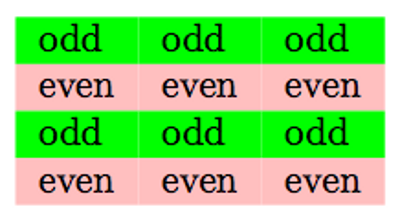## Find the Odd & Even numbers in an Array in C language With Example

C Language program to find the Odd & Even numbers in an Array. This sample C source code is coming under the part of C beginners guide. This simple code can be used for students and professionals.

FIND THE ODD AND EVEN NUMBERS IN ARRAYFind the number of odd and even numbers in an array value by C program is used to calculate the number of odd values and number of even values in a given array. Calculation is made by using the conditional and looping statement. It will separate the both values easily.

//Header files
#include<stdio.h>
#include<conio.h>

void main()
{
//Program variable
int a,n,n,se=0,so=0;

clrscr(); //Function to clear previous output
for(int i=0;i<=9;i++) //Looping statement
{
printf("Enter the number."); //Display function
scanf("%d", n[i]); //Getting input function
}
for(i=0;i<=9;i++)
{
if(n[i]%2=0//Conditional statement
{
se=se+n[i];
}
else
{
so=so+n[i];
}
}
printf("Sum of odd number %d & sum of even number %d", se, so);
getch();
}

#### Post a comment

Note: only a member of this blog may post a comment.

## Labels

Designed By Blogger Templates